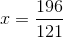## Example Questions

### Example Question #1 : How To Add Square Roots

If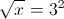what is?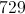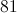Explanation:

Square both sides:

x = (32)2 = 92 = 81

### Example Question #2 : How To Add Square Roots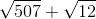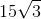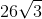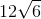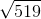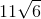Explanation:

To simplify, break down each square root into its component factors: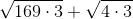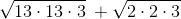You can remove pairs of factors and bring them outside the square root sign. At this point, since each term shares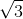, you can add them together to yield the final answer: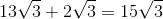### Example Question #1 : Square Roots And Operations

Simplify: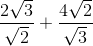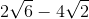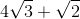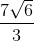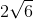Explanation:

Take each fraction separately first:

(2√3)/(√2) = [(2√3)/(√2)] * [(√2)/(√2)] = (2 * √3 * √2)/(√2 * √2) = (2 * √6)/2 = √6

Similarly:

(4√2)/(√3) = [(4√2)/(√3)] * [(√3)/(√3)] = (4√6)/3 = (4/3)√6

√6 + (4/3)√6 = (3/3)√6 + (4/3)√6 = (7/3)√6

### Example Question #4 : How To Add Square Roots

Simplify the following expression: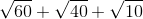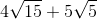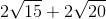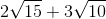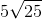Explanation:

Begin by factoring out each of the radicals: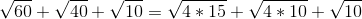For the first two radicals, you can factor out a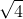or: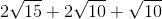The other root values cannot be simply broken down. Now, combine the factors with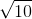: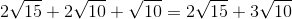### Example Question #1 : How To Add Square Roots

Solve for.

Note,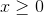: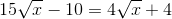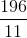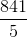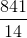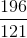Explanation:

Begin by getting yourterms onto the left side of the equation and your numeric values onto the right side of the equation: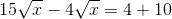Next, you can combine your radicals. You do this merely by subtracting their respective coefficients: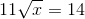Now, square both sides: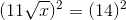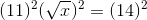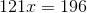Solve by dividing both sides by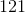: